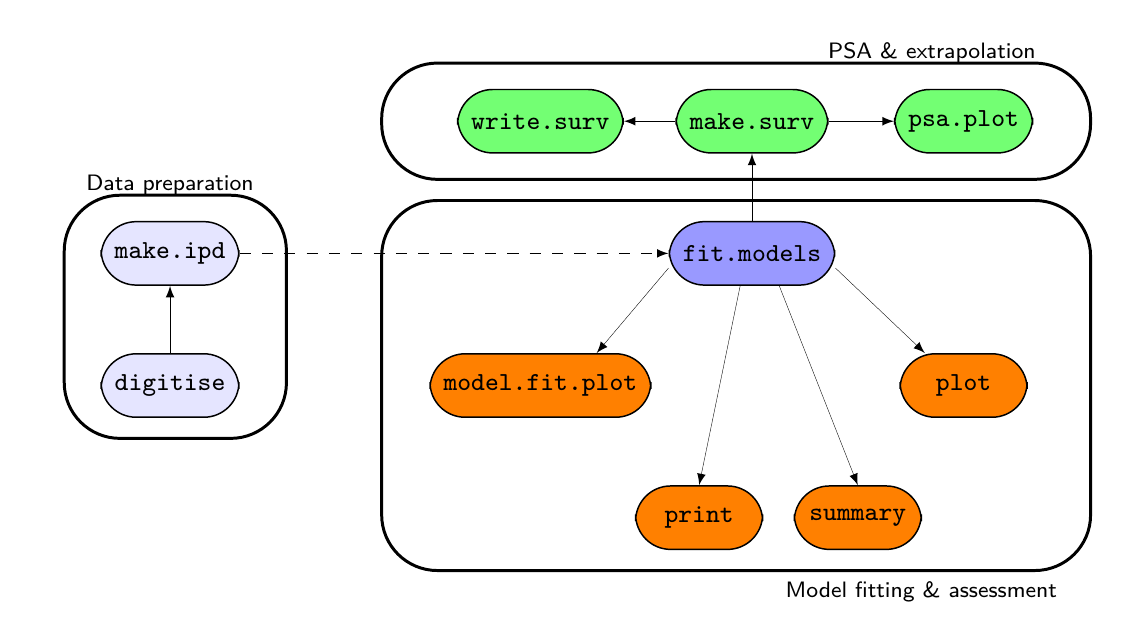# survHE: Survival analysis in health economic evaluation

## Survival analysis in health economic evaluation

Contains a suite of functions to streamline systematically the workflow involving survival analysis in health economic evaluation. survHE can fit a large range of survival models using both a frequentist approach (by calling the R package flexsurv) and a Bayesian perspective. For a selected range of models, both Integrated Nested Laplace Integration (via the R package INLA) and Hamiltonian Monte Carlo (HMC; via the R package rstan) are possible. HMC models are pre-compiled so that they can run in a very efficient and fast way. In addition to model fitting, survHE provides a set of specialised functions, for example to perform Probabilistic Sensitivity Analysis, export the results of the modelling to a spreadsheet, plotting survival curves and uncertainty around the mean estimates.

survHE can take care of the following modelling aspects:

• Reconstruct individual level dataset from digitised data (e.g. from Kaplan-Meier curves)
• Analyse datasets using a hybrid of R formula and specialised commands, i.e. fit.models, which allow the user to select the inferential engine required (mle, inla or hmc), for a range of parametric models (as suggested e.g. by NICE guidelines)
• Perform Probabilistic Sensitivity Analysis directly on the computed parametric survival curves
• Export the output of the statistical model to e.g. a spreadsheet, to complete the economic evaluation (e.g. using Markov models) — of course this step is not necessary and the whole analysis can be embedded in a much bigger (Bayesian) model and performed directly in R!A schematic representation of the main survHE functions and how they interact

# Loads the package
library(survHE)
# Loads some data (Breast Cancer from the package 'flexsurv')
data(bc)
# Fits a model with treatment arm ('group') as the only covariate and
# accounting for censoring ('censrec') using MLE, INLA or HMC
mle=fit.models(formula=Surv(recyrs,censrec)~group,data=bc,distr="exp",method="mle")
inla=fit.models(formula=Surv(recyrs,censrec)~group,data=bc,distr="exp",method="inla")
hmc=fit.models(formula=Surv(recyrs,censrec)~group,data=bc,distr="exp",method="hmc")
# Now prints & plots the output
print(mle); print(inla); print(hmc)
plot(MLE=mle,INLA=inla,HMC=hmc,add.km=TRUE,lab.profile=c("Good","Medium","Poor"))Model fit for the Exponential model, obtained using Flexsurvreg
(Maximum Likelihood Estimate). Running time: 0.037 seconds

mean         se      L95%      U95%
rate        0.0603838 0.00845542 0.0458911 0.0794534
groupMedium 0.8180219 0.17122084 0.4824352 1.1536086
groupPoor   1.5375232 0.16280169 1.2184378 1.8566087

Model fitting summaries
Akaike Information Criterion (AIC)....: 1668.212
Bayesian Information Criterion (BIC)..: 1681.805

Model fit for the Exponential model, obtained using INLA (Bayesian inference via
Integrated Nested Laplace Approximation). Running time: 0.79385 seconds

mean         se      L95%      U95%
rate        0.0610699 0.00873326 0.0450402 0.0783935
groupMedium 0.8107048 0.17083145 0.5003443 1.1368302
groupPoor   1.5294877 0.15669343 1.2410091 1.8446508

Model fitting summaries
Akaike Information Criterion (AIC)....: 1668.192
Bayesian Information Criterion (BIC)..: 1681.737
Deviance Information Criterion (DIC)..: 1668.192

Model fit for the Exponential model, obtained using Stan (Bayesian inference via
Hamiltonian Monte Carlo). Running time: 4.2649 seconds

mean         se      L95%    U95%
rate        0.060283 0.00837485 0.0445859 0.07878
groupMedium 0.825360 0.17463171 0.4688271 1.16799
groupPoor   1.546096 0.16208830 1.2348947 1.86399

Model fitting summaries
Akaike Information Criterion (AIC)....: 1670.223
Bayesian Information Criterion (BIC)..: 1688.347
Deviance Information Criterion (DIC)..: 1668.225

A full documentation (published in the Journal of Statistical Software) is available here.

## Installation

There are two ways of installing survHE. A “stable” version (as of 7 October 2020, it is on version 1.1.1) is packaged and binary files are available for Windows and as source. To install the stable version from CRAN, run the following commands

install.packages("survHE")

This process can be quite lengthy, if you miss many of the relevant packages. Also, the pre-compiled rstan models do take some time at installation (but this steps produces substantial savings at compilation and running time!).

survHE is also available from the GitHub repository. The master branch is the same as the official one, hosted on CRAN. You can still install it from GitHub using the following commands on the R terminal. On a Windows machine:

pkgs <- c("flexsurv","Rcpp","rms","xlsx","rstan","INLA","Rtools","devtools","dplyr","ggplot2")
install.packages(pkgs,repos=repos,dependencies = "Depends")

before installing the package using devtools:

devtools::install_github("giabaio/survHE")

Under Linux or MacOS, it is sufficient to install the package via devtools:

install.packages("devtools")
devtools:install_github("giabaio/survHE")

Finally, there is a development version, which is stored in the devel branch of the GitHub repository. This version is continuously updated (and we welcome comments and suggestions - you can open an “Issue” here). The process for installation is essentially the same as above with the only final difference

devtools:install_github("giabaio/survHE", ref="devel")

(the option ref="devel" instructs R to look for the relevant files in the branch named devel).

We recommend using the GitHub installation from the master repository as it will fix minor issues that may become known quicker than the stable version on CRAN.

### Installation issues

Installation of the development version via devtools:install_github() can fail in a MS Windows environment with the following error message:

Error in .shlib_internal(args) : C++14 standard requested but CXX14 is not defined

This is due to known issues (see for example here) with new(er) versions of rstan (which survHE uses for full Bayesian modelling). rstan uses by default version 14 of the C++ compiler, so R needs to know and act accordingly. This can be solved by running the following code

dotR <- file.path(Sys.getenv("HOME"), ".R")
if (!file.exists(dotR))
dir.create(dotR)
M <- file.path(dotR, "Makevars.win")
if (!file.exists(M))
file.create(M)
cat("\nCXX14FLAGS=-O3 -Wno-unused-variable -Wno-unused-function",
"CXX14 = $(BINPREF)g++ -m$(WIN) -std=c++1y",
"CXX11FLAGS=-O3 -Wno-unused-variable -Wno-unused-function",
file = M, sep = "\n", append = TRUE)
Last updated: Monday 17 January 2022

## Relevant publications

Baio, Gianluca. 2020. “survHE: Survival Analysis for Health Economic Evaluation and Cost-Effectiveness Modeling.” Journal of Statistical Software, Articles 95 (14): 1–47. https://doi.org/10.18637/jss.v095.i14.